Future Energy Demand in Lao PDR

Dr. Kazuhiro Fukuyo

Professor, Yamaguchi University

(Published: May 13th, 2010)

1.      Introduction

The empirical relationships between energy demand and economic growth have been examined by many papers. However, there are remarkably few papers which study the relationship between energy demand and economic growth in Lao PDR, although the country holds important position in the electricity supply in Southeast Asia. In this short communication, the author shows the longitudinal relationship between energy demand and GDP in Lao PDR and discusses the future energy demand.

2.      Energy demand and supply in Lao PDR

Figures 1 and 2 show the longitudinal trends of energy production and consumption in Lao PDR, which are based on the data provided by U.S. Energy Information Administration . These data  are described in various units. Therefore, Table 1 was used to convert the energy units.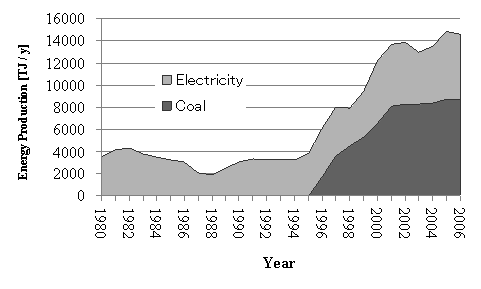Fig. 1 Energy production in Lao PDR

(Data of coal production is not available before 1995)

Table 1 Units of measurement

 Volume and Weight Calorific values 1 barrel = 158.987295 liters 1 short ton = 907.18474 kg Petroleum (crude oil), 1 litter: 38.2 MJ Coal, 1kg: 28.9 MJ Electricity (consumption), 1kWh: 3.6MJ

Figure 1 shows that only coal and electricity are main energy sources produced in Lao PDR. Electricity is mainly produced by the hydroelectric power stations. The volume of electricity production increased 1.7 times between 1980 and 2006, and the volume of coal production increased 4.9 times between 1996 and 2006.

Figure 2 shows petroleum, coal, and electricity consumption. The volumes of electricity and petroleum consumption increased 4.1 and 2.6 times, respectively, between 1981 and 2006. Electricity consumption grew significantly during this decade. This growth is thought to be caused by the widespread use of electric home appliances. According to a socio-economic atlas, only 58% percent of households can access the electricity, but 50% of total electricity is used in households for lighting, refrigerators, television, radio, etc. Spread of air-conditioners also should be taken into account.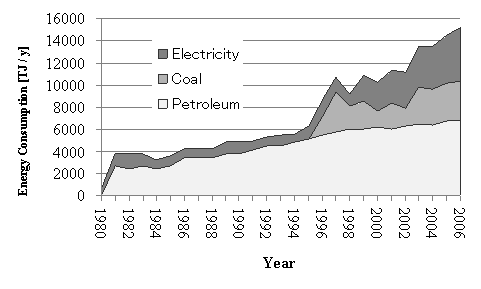Fig. 2 Energy consumption in Lao PDR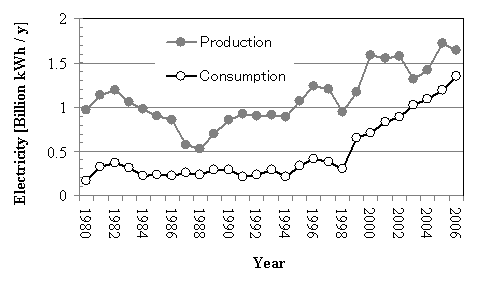Fig. 3 Comparison between electricity production and consumption

Figure 3 shows comparison between electricity production and consumption. The electricity consumption grows faster than the production. Therefore, the exportable electricity, i.e., the difference between production and consumption is becoming smaller and smaller in these years. The growth of electricity demand is now considered an important issue.

3.      Electricity demand and economic growth

It is empirically known that there is a relationship between economic growth and increase in electricity demand. An example for the relationship is shown in Fig. 4. Real GDP (Gross domestic product at constant market prices in 1995) and electricity consumption of Japan between 1955 and 2002  show the strong linear relationship (The coefficient of determination, R2 is very high).

What about the relationship in Lao PDR? Figure 5 shows Real GDP (at constant market prices in 1995)  and electricity consumption of Lao PDR between 1980 and 2006.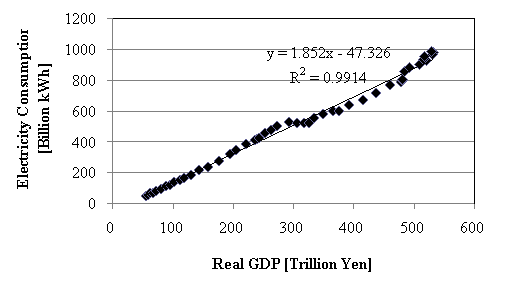Fig. 4 Real GDP and Electricity consumption in Japan (1955 – 2002)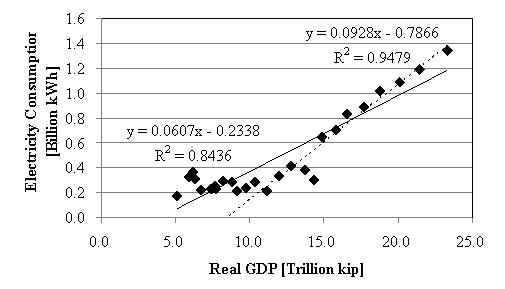Fig. 5 Real GDP and Electricity consumption in Lao PDR (1980 – 2006)

Now we assumed the following regression formula for the electricity consumption in Lao PDR.

y
= a x + b           (1)

where y is the yearly electricity consumption [billion kWh], x is the real GDP [trillion kip], and a and b are the coefficients.

The regression analysis on the all data gives the following regression formula:

y = 0.0607 x-– 0.2338       (2)

The regression line is a solid one in Fig. 5. The regression coefficient, R2 is 0.8346. However, many of the data points are away from the regression line.

The regression analysis on the data after 1993, the year that the real GDP exceeded 10 trillion kip, gives the following regression formula:

y = 0.0928 x -– 0.7866       (3)

The regression line is a broken one in Fig. 5. The regression coefficient, R2 is 0.9479 and higher than that of Eq. (2). The difference of these regression results indicates that the relationship between the real GDP and electricity consumption was changed after the 1990s.

4.      Future electricity demand

IMF predicted the future real and nominal GDP of Lao PDR as shown in Table 2 . By using this data and the regression formula (3), we can predict the future electricity demand as shown in Fig. 6 (White circles are predicted points).

Table 2 Future real and nominal GDPs of Lao PDR predicted by IMF 

 Year Real GDP [billion kip] Nominal GDP [billion kip] 2009 2010 2011 2012 2013 2014 2015 29156 31263 33574 35823 38487 41345 45129 47567 53069 59606 66040 73661 81612 91730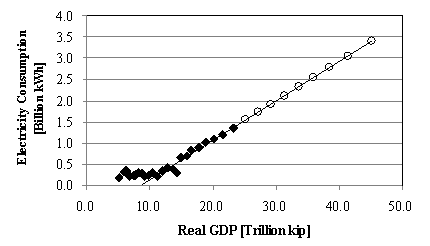Fig. 6 Predicted real GDP and electricity consumption in Lao PDR (2009 – 2015)

This prediction is very simple. However, it is considered to be rational that the future electricity demand in Lao PDR will increase with the economic growth. If we carry out more precise prediction, we should take the following factors into account:

·          Improvement of the grid power system

·          Supply capacity of electricity

·          Spread of electric home appliances

The situation of the grid system and the supply capacity is constraint and the spread of electric appliances is the increasing factor. For example, the government tries to raise the electrification rate to 90% by 2020, according to the atlas . This will ease the constraint. Nam Theun 2 Hydroelectric Project will strengthen the supply capacity. This will also relax the constraint.

5.      Conclusion

In this short communication, the longitudinal changes in energy supply and demand in Lao PDR are presented. The simple regression analysis showed the strong relationship between the real GDP and electricity consumption. Based on the regression formula and the future GDP values predicted by IMF, the future electricity demand is predicted.

References

 U.S. Energy Information Administration: Laos Energy Profile,

http://tonto.eia.doe.gov/country/country_energy_data.cfm?fips=LA, Last Update: January 6, 2010

 Department of Statistics Ministry of Planning and Investment Government of the Lao PDR; Swiss National Centre of Competence in Research North-South Switzerland; Centre for Development and Environment, University of Bern; Lao National Mekong Committee Secretariat, Water Resources and Environmental Administration, Prime Minister's Office Government of the Lao PDR, Socio-economic ATLAS of the Lao PDR, 2008 (ISBN: 978-3-905835-03-8)

 Statistic Bureau, Ministry of Internal Affairs and Communications, Japan: Historical Statistics of Japan, http://www.stat.go.jp/english/data/chouki/index.htm, 2008

 IMF: Data and Statistics, http://www.imf.org/external/data.htm, Last Updated: April 30, 2010

<back>